# AP2 Unit 1: vPython Modeling

To open the AP2 course, the students will learn how to make a computer simulation using vPython over about 2 weeks of classes. I would like to thank John Burk’s awesome series of blogs. I’m also pulling from resources found in the additional materials from the AMTA website.

The first part of the unit is learning some of the basics of computer programming, how to create objects, change attributes, etc. The fun begins when they learn how to make an object move in one dimension. They begin to see how the physics we learned in the AP 1 course is used in the code. After learning how to make a sphere move, they quickly proceed on to make that ball bounce with a perfectly elastic collision off a wall. From their, they add a wall on the opposite side to make the ball bounce back and forth. Then they learn how to adjust the code so that the ball bounces inside a box with motion in all three dimensions.

The next big step in the sequence is to add a second ball. To start off, we go back to 1D motion. They learn how to have the balls bounce with a perfectly elastic collision using a transformation into the Center of Momentum frame of reference to make the calculation easier. After that hurdle, we then move on to two balls moving in three dimensions. Once they can successfully do that, I ask the students what they would need to do to add a third ball. By this point (about a week into programming), they can already realize that they would need to copy the code for the ball interaction twice (ball 1 to ball 2, ball 1 to ball 3, and ball 2to ball 3).

Before they start doing that, I introduce lists to help clean up the code. They create a list for walls, and a second list of balls. Once I help them figure out how to write the math for the interactions between lists and among members of the list of balls, we can now start adding a lot of balls quickly.

The last step is to start making the code able to do the unit’s experiment. They set up parameters at the beginning of the code to input the size of the box, the average velocity of the balls, and the exact number of balls. For each one, I introduce the ability to create random numbers. For velocity, I also introduce spherical coordinates. They create a random velocity based on a set mean and standard deviation (random gaussian number), with random angles, then transform those into the x, y, and z components of the velocity for the code. I also show them how to have the code output the average kinetic energy of the balls, the total kinetic energy, and the pressure the balls exert as they bounce off the walls.

At first the lab groups are to vary 1 input parameter and record the other inputs parameters and the output results. As you begin running the program, and manipulating the given inputs, you can generate graphs from them.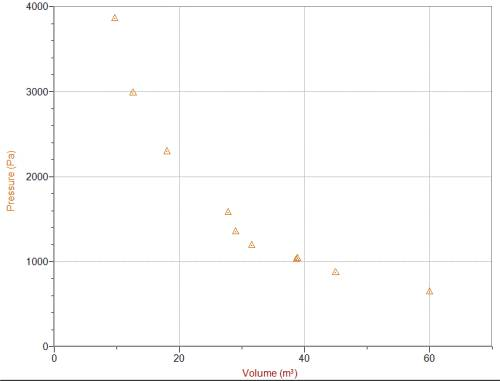We then define temperature as the average kinetic energy of the particles to create a Pressure vs. Temperature graph: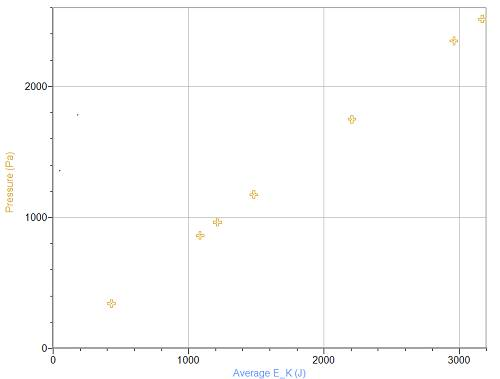From the three graphs we can see that pressure is proportional to number of balls and Temperature, and inversely proportional to volume. If we then graph pressure vs. NT/V we get:We can then conclude create an equation:$P = k\frac{NT}{V}$

I haven’t figured out how I need to tweak to code such that the slope in Boltzmann’s constant,$k_b$, but I’m guessing is has something to do with choosing the correct parameters. You can then discuss that the$SI$ units for counting particles is the mole. This changes the above equation to:$P = \frac{k}{N_A} \frac{nT}{V}$

Which can be simplified as:$P = \frac {nRT}{V}$

You can also look at which variables effect the total energy of the system. The graphs that show something are: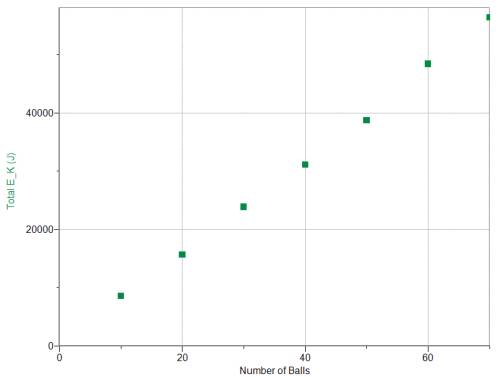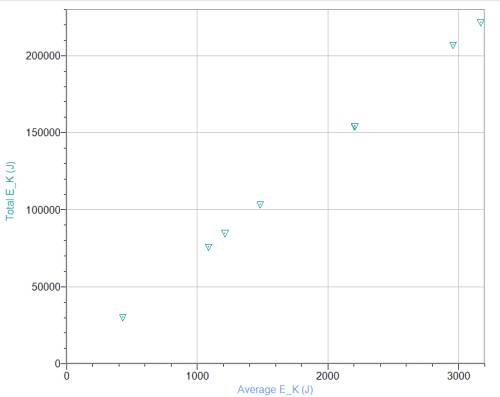If we then plot Total Energy vs NT you get the following: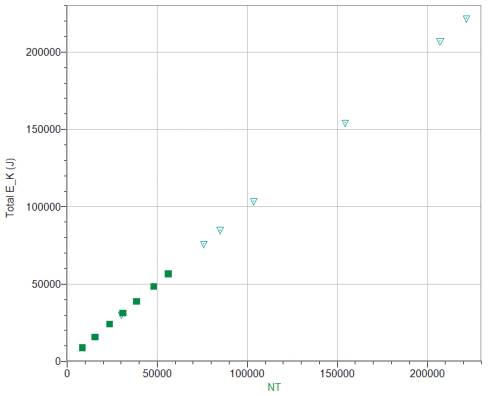If you then discuss the units of each of the combined graphs ($P$ vs.$\frac{NT}{V}$ and$E_{total}$ vs.$NT$) you will see that the units of slope are the same. If you then make a new graph of$E_{total}$ vs.$kNT$, where$k$ is the slope from the$P$ vs.$\frac {NT}{V}$ graph, you get the following:From this graph we can see that the slope is very close to$\frac {3}{2}$, so we can create the equation:$E_{total} = \frac {3}{2} kNT$

Since the only flavor of energy present in our system we can say that the total energy of the particles$E_{total}$ is the  of the system$U$. Thus:$U = \frac{3}{2}kNT$

Since I had to do this in a computer lab, I did all of this before we moved into the lab to begin the second unit, the System of Ideal Particles Model. If you have computers in the class room, you might want to delay discussing the combined graphs and subsequent equations until after completing the paradigm experiments.

Advertisements